# RMS Error

## Producer Field Guide

HGD_Product
Producer Field Guide
HGD_Portfolio_Suite
Producer

RMS error is the distance between the input (source) location of a GCP and the retransformed location for the same GCP. In other words, it is the difference between the desired output coordinate for a GCP and the actual output coordinate for the same point, when the point is transformed with the geometric transformation.

RMS error is calculated with a distance equation: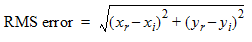Where:

xi and yi are the input source coordinates

xr and yr are the retransformed coordinates

RMS error is expressed as a distance in the source coordinate system. If data file coordinates are the source coordinates, then the RMS error is a distance in pixel widths. For example, an RMS error of 2 means that the reference pixel is 2 pixels away from the retransformed pixel.

Residuals and RMS Error Per GCP

The GCP Tool contains columns for the X and Y residuals. Residuals are the distances between the source and retransformed coordinates in one direction. They are shown for each GCP. The X residual is the distance between the source X coordinate and the retransformed X coordinate. The Y residual is the distance between the source Y coordinate and the retransformed Y coordinate.

If the GCPs are consistently off in either the X or the Y direction, more points should be added in that direction. This is a common problem in off-nadir data.

RMS Error Per GCP

The RMS error of each point is reported to help you evaluate the GCPs. This is calculated with a distance formula: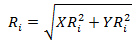Where:

Ri = RMS error for GCPi

XRi = X residual for GCPi

YRi = Y residual for GCPi

The figure below illustrates the relationship between the residuals and the RMS error per point.

Residuals and RMS Error Per Point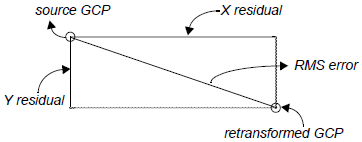Total RMS Error

From the residuals, the following calculations are made to determine the total RMS error, the X RMS error, and the Y RMS error:

Where:

Rx = X RMS error

Ry = Y RMS error

T = total RMS error

n = the number of GCPs

i = GCP number

XRi = X residual for GCPi

YRi = Y residual for GCPi

Error Contribution by Point

A normalized value representing each point’s RMS error in relation to the total RMS error is also reported. This value is listed in the Contribution column of the GCP Tool.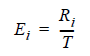Where:

Ei = error contribution of GCPi

Ri = RMS error for GCPi

T = total RMS error

Tolerance of RMS Error

In most cases, it is advantageous to tolerate a certain amount of error rather than take a more complex transformation. The amount of RMS error that is tolerated can be thought of as a window around each source coordinate, inside which a retransformed coordinate is considered to be correct (that is, close enough to use). For example, if the RMS error tolerance is 2, then the retransformed pixel can be 2 pixels away from the source pixel and still be considered accurate.

RMS Error Tolerance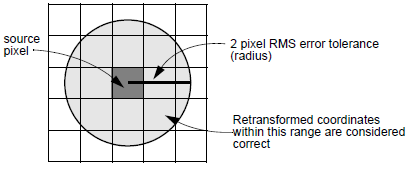Acceptable RMS error is determined by the end use of the data base, the type of data being used, and the accuracy of the GCPs and ancillary data being used. For example, GCPs acquired from GPS should have an accuracy of about 10 m, but GCPs from 1:24,000-scale maps should have an accuracy of about 20 m.

It is important to remember that RMS error is reported in pixels. Therefore, if you are rectifying Landsat TM data and want the rectification to be accurate to within 30 meters, the RMS error should not exceed 1.00. Acceptable accuracy depends on the image area and the particular project.

Evaluating RMS Error

To determine the order of polynomial transformation, you can assess the relative distortion in going from image to map or map to map. One should start with a 1st-order transformation unless it is known that it does not work. It is possible to repeatedly compute transformation matrices until an acceptable RMS error is reached.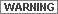Most rectifications are either 1st-order or 2nd-order. The danger of using higher order rectifications is that the more complicated the equation for the transformation, the less regular and predictable the results are. To fit all of the GCPs, there may be very high distortion in the image.

After each computation of a transformation and RMS error, there are four options:

• Throw out the GCP with the highest RMS error, assuming that this GCP is the least accurate. Another transformation can then be computed from the remaining GCPs. A closer fit should be possible. However, if this is the only GCP in a particular region of the image, it may cause greater error to remove it.
• Tolerate a higher amount of RMS error.
• Increase the complexity of transformation, creating more complex geometric alterations in the image. A transformation can then be computed that can accommodate the GCPs with less error.
• Select only the points for which you have the most confidence.